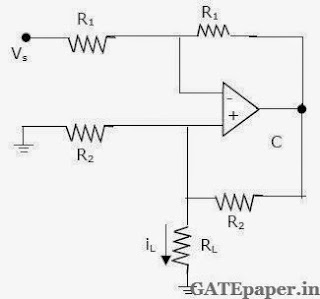### GATE 2004 ECE Video Solutions on Analog Circuits (Analog Electronics)

1. An ideal OP-AMP is an ideal
a. Voltage Controlled Current Source
b. Voltage Controlled Voltage Source
c. Current Controlled Current Source
d. Current Controlled Voltage Source

2. Voltage series feedback (also called series-shunt feedback) results in
a. Increase in both input and output impedances
b. Decrease in both input and output impedances
c. Increase in input impedance and decrease in output impedance
d. Decrease in input impedance and increase in output impedance

3. The circuit in the figure is aa. Low pass filter
b. High pass filter
c. Band pass filter
d. Band reject filter

4. A bipolar transistor is operating in the active region with a collector current of 1 mA. Assuming that the β of the transistor is 100 and the thermal voltage (VT) is 25 mV. The Transconductance (gm) and the input resistance (rπ) of the transistor in the common emitter configuration are
a. gm = 25 mA/V and rπ = 15.625 KΩ
b. gm = 40 mA/V and rπ = 4.0 KΩ
c. gm = 25 mA/V and rπ = 2.5 KΩ
d. gm = 40 mA/V and rπ = 2.5 KΩ

5. the value of capacitor C required for sinusoidal oscillations of frequency 1 kHz in the circuit of the figure is6. in the OP-AMP circuit given in the figure, the load current iL isa. – VS / R2
b. VS / R2
c. – VS / RL
d. VS / R1

7. In the full wave rectifier using two ideal diodes, Vdc and Vm are the dc and peak values of the voltage respectively across a resistive load. If PIV is the peak inverse voltage of the diode, then the appropriate relationships for this rectifier are